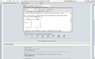# Analyzing circuits with resistor/battery

scholio

## Homework Statement

use circuit shown:

if my 'drawing' gets reformatted incorrectly, this is what it 'looks' like in words.
a 25 volt battery connected to a 16ohm at the top which connects at a junction to a 8ohm that goes down and a 1 ohm that goes to the right. the 1 ohm forms top right corner with a 3 ohm resistor pointing down to the bottom left corner. the bottom left corner connects to a4 ohm resistor that connects with 8 ohm resistor coming down from the 16 ohm - 1 ohm wire. the 4 ohm is connected at a junction to a 5 ohm resistor which connects to the bottom of the battery.

the battery, 8ohm, and 3 ohm are parallel. the 16ohm and 1 ohm wire is parallel to the 5ohm-4ohm wire. both are perpendicular to the battery, 8ohm and 3 ohm resistor.

btw, for future reference, what is the best way to put up diagrams like circuit so that it won't get messed up during posting?

_____16ohm__________1ohm___
| | |
--- | |
25volts 8 3
- o o
| h h
| m m
|____5ohm__|________4ohm___|

a) what is the potential difference accross the 4 ohm resistor in the circuit?
b) what is the current in the 8 ohm resistor?
c) at what rate is the energy dissipated in the 16 ohm resistor and what fractions of all the energy dissipated is this?

## Homework Equations

series resistors R = R_1 + R_2 + R_3 ...

parallel resistors 1/R = 1/R_1 + 1/R_2 + 1/R_3 ...

V = IR where V is potential, I is current, R is resistance

current I = epsilon/(R_1 + R_2) ---> not sure what epsilon stands for? R is resistor

## The Attempt at a Solution

i want to know the best way to handle the circuit, i am not sure what to make into series or parallel resistors to simplify the circuit.

especially how to handle the 8 and 3 ohm resistors in the center

help appreciated

#### Attachments

•circ.JPG
40.3 KB · Views: 489
Last edited:

Staff Emeritus
Gold Member
There are 3 resistors that are obviously in series (that is, the same current flows through all 3 of them). Can you spot them?

scholio
the 16 ohm, 1 ohm and 3 ohm resistors?

if i made those three in series then how would the 8 ohm and 4 ohm be involved when i redraw the circuit?

attach a screen shot because my 'drawing' got messed up..if it helps

Last edited:
Staff Emeritus
Gold Member
No, the 16 ohm resistor is clearly not in series with the others. The current flowing through that resistor gets split off into the 8 ohm resistor the branch containing the 1, 3, and 4 ohm resistors.

Try again?

scholio
the 1, 3, 4 ohm resistors? and then the 16, 8, 5 ohm resistors, then both series joined in parallel?

Staff Emeritus